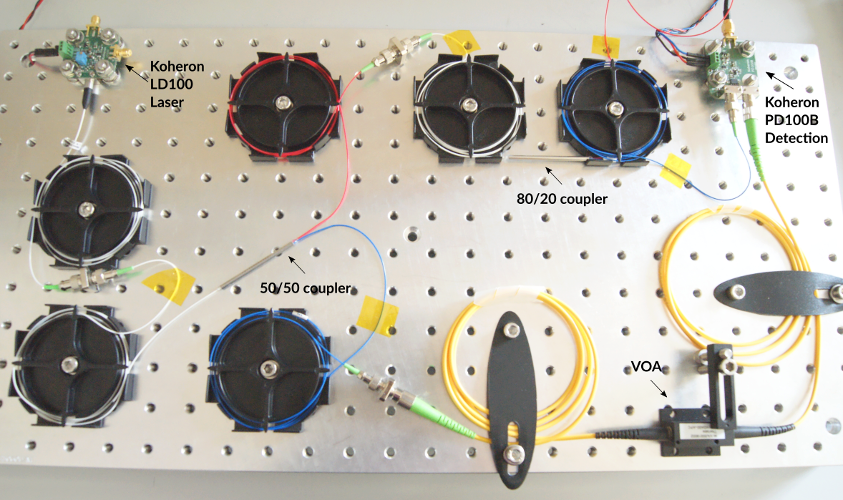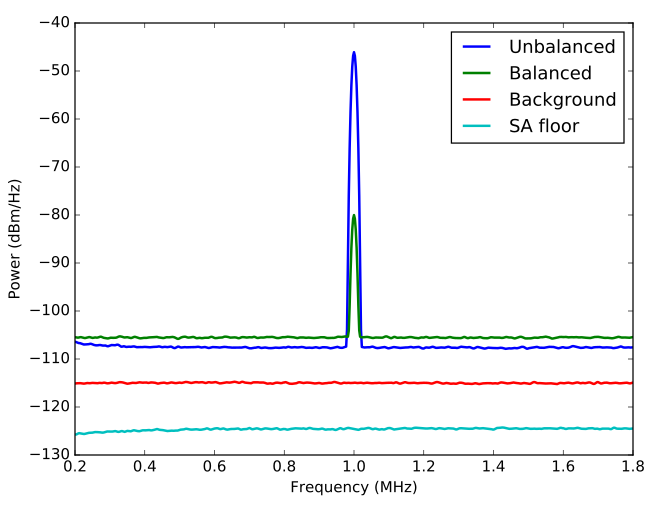# Common mode rejection ratio of a balanced detector

2017-03-13

To reach the ultimate noise limit in a coherent detection setup, it is often necessary to reject the relative intensity noise (RIN) of the laser.

In this post, we will present a setup to measure the common mode rejection ratio (CMRR) of the Koheron PD100B balanced photodetector.A balanced detection subtracts and amplifies the photocurrents of two matched photodiodes as shown below:

The output signal is $V = G (P_{+} - P_{-})$, where $G$ is the detector gain (in V/W), and $P_{+}$ and $P_{-}$ are the optical powers incident on each photodiode. This setup is often used in interferometry, where the two photodiodes detect the the optical power on each arm of the interferometer. This allows rejecting the laser intensity noise while keeping the amplitude modulation caused the phase fluctuations that we want to measure.

## Common mode rejection ratio

In practice some residual misbalance within the detector will limit the rejection efficiency. We can model the misbalance with a different detector gain $G_{\pm}$ for each detector. The rejection efficiency is characterized by the CMRR, which is difference between the two gains normalized by the average gain. This can be written in decibels as:

$$\mathrm{CMRR}_{\rm dB} \equiv 10 \log_{10} \left( 2 \frac{G_{+} - G_{-}}{G_{+} + G_{-}} \right).$$

## Measurement

If the two photodiodes are illuminated with the same optical power $P$, the balanced signal is $V_{\rm bal} = (G_{+} - G_{-}) P$. When one of the input is removed from the detector, the unbalanced signal is $V_{\rm unbal} = G_{+} P$. Assuming $G_{+} \simeq G_{-}$, we get:

$$\mathrm{CMRR}_{\rm dB} \simeq 10 \log_{10} \left( \frac{G_{+} - G_{-}}{G_{+}} \right) = 10 \log_{10} \left( \frac{V_{\rm bal}}{V_{\rm unbal}} \right).$$

We use the following setup to measure the ratio between the balanced and unbalanced signals:A Koheron LD100 laser is modulated with a sinusoidal signal of 1 MHz frequency and 30.4 mVpp amplitude using a waveform generator (Keysight 33600A). The optical power is split into two equal parts. To tune the relative power between the two parts a variable optical attenuator (VOA) is inserted in one arm, and 20 % of the other arm power is used. Since a delay limits the achievable CMRR, we properly adjust the length difference between the two arms. The output of the PD100B balanced photodetector is directly connected to a spectrum analyzer (Textronix RSA306). The 1 MHz signal is measured on the spectrum analyzer in the balanced case (when the two powers are equal to 395 µW), and in the fully unbalanced case (with only one input connected). We obtain the following result:As expected, the peak amplitude is reduced in the balanced configuration. We also observe that the noise floor is higher in the balanced case, this is because the total incident power is doubled compared to the unbalanced case resulting in a 3 dB excess shot-noise.

By computing the ratio between the peak amplitudes in the balanced and unbalanced case, we find that the CMRR of the PD100B balanced photodetector is 35.8 dB at 1 MHz.